# Magnitude & Direction of the Electric Force on a Point Charge

An error occurred trying to load this video.

Try refreshing the page, or contact customer support.

Coming up next: Coulomb's Law: Variables Affecting the Force Between Two Charged Particles

### You're on a roll. Keep up the good work!

Replay
Your next lesson will play in 10 seconds
• 0:03 Magnitude & Direction
• 1:44 Two Point Charges
• 2:47 Point Charges in a Line
• 4:31 Point Charges in a 2D Plane
• 5:44 Lesson Summary

Want to watch this again later?

Timeline
Autoplay
Autoplay
Speed

#### Recommended Lessons and Courses for You

Lesson Transcript
Instructor: Betsy Chesnutt

Betsy teaches college physics, biology, and engineering and has a Ph.D. in Biomedical Engineering

Coulomb's law can be used to calculate the electric force between two point charges, but what do you do when there are more than two charges present? In this lesson, you'll learn how to calculate the magnitude and direction of the electric force when multiple charges are present.

## Magnitude & Direction

When you hold a ball up above the ground and let it go, you know that it will fall toward the earth. This happens because the earth exerts an attractive force on the ball, causing it to move toward the earth, speeding up as it gets closer.

Something similar happens when you hold two charges near each other and let them go. The charges will exert electric forces on each other, causing them to either move apart or come together. However, unlike the gravitational force exerted by the earth on the ball, which is always an attractive force, the electric force between two point charges can be either attractive or repulsive, depending on the type of charges involved.

If the two charges are different, with one being positive and one negative, then they will attract each other. But, if the two charges are the same, with both being either positive or negative, then they will repel each other.

Coulomb's Law says that the magnitude of the electric force between two charged objects is directly proportional to the charge on each object (symbolized by q1 and q2) and inversely proportional to the distance between the charges (r). Therefore, you can use the following equation to calculate the magnitude of the force between ANY two charged objects: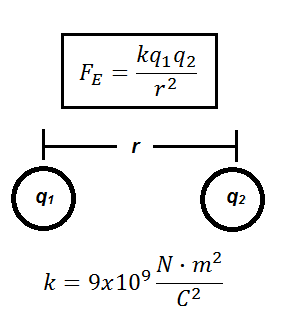Remember that this will only give you the magnitude of the force, and not the direction, so you should use the absolute value of q1 and q2. The magnitude of a force will never be a negative number!

## Two Point Charges

Let's first try to use Coulomb's Law to calculate the magnitude and direction of the electric force on a point charge when there are only two point charges present.

In the situation shown here, what is the magnitude and direction of the force exerted on the charge on the left by the charge on the right?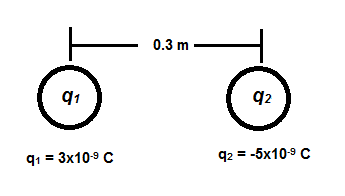First, determine the direction of the force on q1. Since one charge is negative and the other is positive, the charges will exert attractive forces on each other. Therefore, the electric force on q1 will be directed toward the right.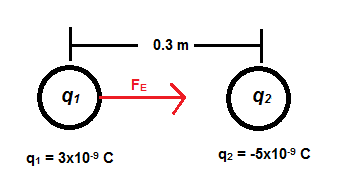Next, use Coulomb's Law to calculate the magnitude of the force, like this: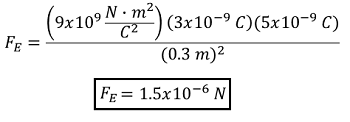Therefore, the electric force exerted on q1 is 1.5x10-6 N directed towards the right.

## Point Charges in a Line

Next, let's try to find the net force on a charge when more than one electric force is exerted on it, as shown here. What is the net force on q1 now?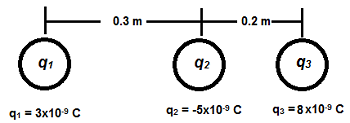In this situation, both q2 AND q3 exert electric forces on q1. To find the net force on q1, first determine the direction of the force that each of the other charges will exert on it. As before, q2 will exert an attractive force to the right, which we already calculated. Because q1 and q3 are both positively charged, then q3 will exert a repelling force on q1 that will be directed toward the left, like this: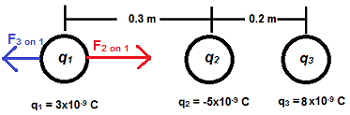Then, use Coulomb's Law to calculate the magnitude of each force. We already know the magnitude of F2 since we calculated it in the first example, so we just need to find F3: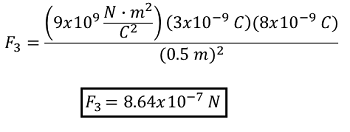To unlock this lesson you must be a Study.com Member.

### Register to view this lesson

Are you a student or a teacher?

### Unlock Your Education

#### See for yourself why 30 million people use Study.com

##### Become a Study.com member and start learning now.
Back
What teachers are saying about Study.com

### Earning College Credit

Did you know… We have over 160 college courses that prepare you to earn credit by exam that is accepted by over 1,500 colleges and universities. You can test out of the first two years of college and save thousands off your degree. Anyone can earn credit-by-exam regardless of age or education level.